# Python if-else: The Complete Guide

Python supports the usual logical conditions in mathematics. The decision-making process is required when we want to execute code only if a specific condition is satisfied. The conditional if..elif..else statement is used in the Python programming language for decision-making.

There come situations in real life when we need to make some decisions, and based on those decisions, we decide what we should do next. Similar situations arise in programming also where we need to make some decisions, and based on this decision, we will execute the next block of code.

## Python if-else

Python if-else is a built-in logical statement that performs different computations or actions depending on whether the specific boolean constraint evaluates to true or false.

Conditional statements are handled by the if statements in Python.

### Syntax

There can be zero or more elif parts, and the other part is optional.

The keyword elif is short for “else if” and is useful to avoid excessive indentation.

```if expression
Statement
else
Statement```

Here, the program evaluates the expression and will execute statement(s) only if the text expression is True. If the text expression is False, the else statement is not executed.

In Python language, the body of the if indentation indicates a statement. The body starts with an indentation, and the first unindented line marks the end.

Python interprets non-zero values as True. None and 0 are interpreted as False.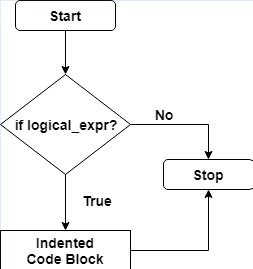## Example of Python if

```# app.py

data = 2
if data > 0:
print(data, "is a positive number.")
print("This is always printed.")

data = -2
if data > 0:
print(data, "is a positive number.")
print("This is also always printed.")```

See the below output.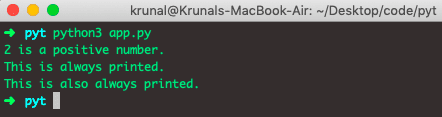In the code, we have defined a variable data with value 2. Now, we have checked the value of the data variable, and if it is > 0, it returns a positive number.

The body of the if statement is executed only if this evaluates to True.

If variable data is equal to -2, the test expression is false, and the body inside the body of if is skipped.

See the code carefully that we have used the two if statements and not else or elif statements.

Also, we have used indentation. So the body statement of the if statement is written with indentation. Indentation in Python language is significant.

You can see after if and body statements; there is another print line without indentation. So, it will always print regardless of the outcome of the if statement.

## Python if…else Statement

### Syntax

```if expression:
body of if
else:
body of else```

The if..else statement evaluates the expression and will execute the body only when the test condition is True.

If the condition is False, the body else is executed. Indentation is used to separate the blocks.

```# app.py

data = -2
if data > 0:
print(data, "is a positive number.")
else:
print(data, "is a negative number.")
```

See the below output.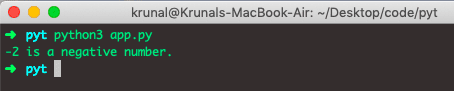In the above example, when data is equal to 2, the test expression is true, the body of if is executed, and the body of else is skipped.

If data is equal to -2, the test expression is false, the body of else is executed, and the body of if is skipped.

If data is > 0, the test expression is true, the body of if is executed, and the body of else is skipped.

There might be many instances when your “else condition” won’t give you the desired result.

It will print out the wrong conclusion as there is a mistake in the programming logic. In most cases, it happens when you have to justify more than two statements or conditions in the program.

## Python if..elif..else Statement

### Syntax

```if expression:
body of if
elif expression:
body of elif
else:
body of else```

The elif is the shorthand for else if. It allows us to check for multiple expressions.

If the condition for the if statement is False, it checks the condition of the next elif block and so on.

If all the conditions are False, the body of the else statement is executed.

Only one block among the several if..elif..else blocks is executed according to the condition.

The if block should have only one else block. But it can have multiple elif blocks.

You need to keep in mind that.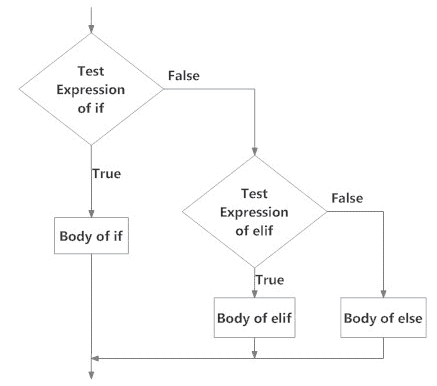### Example of if..elif..else

```# app.py

data = 0
if data > 0:
print(data, "is a positive number.")
elif data == 0:
print("The data is:", data)
else:
print(data, "is a negative number.")
```

See the output below.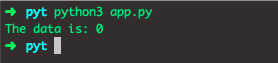When the data variable is positive, a positive number is printed.

If the data is equal to 0, The data is 0 and is printed.

If the data is negative, the Negative number is printed.

In our example, the elif statement will be executed.

## Python Nested if statements

We can have an if statement inside another if..elif..else statement. It is called nesting in computer science and programming.

Any number of those statements can be nested inside one another.

Indentation is the only way to figure out a level of nesting. However, this can get confusing when you have multiple and complex conditions, so it must be avoided if we can.

See the following example.

```# app.py

data = 4
if data > 0:
if data > 3 and data < 5:
print(data, "is my roll number.")
else:
print(data, "is not roll number")
elif data == 0:
print("The data is:", data)
else:
print(data, "is a negative number.")
```

See the below output.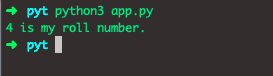So, we have written the nested if..else statement in the if statement. In Python language, it is all about perfect indentation. So, if your indentation and logic are correct, you will surely get your desired output.

Python supports the following logical conditions from mathematics:

1. Equals: a == b
2. Not Equals: a != b
3. Less than: a < b
4. Less than or equal to a <= b
5. Greater than: a > b
6. Greater than or equal to a >= b

The above conditions can be used in several ways, most commonly in the “if statements” and loops.

An “if statement” is written by using the if keyword.

## How to execute a conditional statement with minimal code

In this section, we will see how we can see out the conditional statement. Instead of executing the code for each condition separately, we can use them with a single code.

### Syntax

`X If Y else Z`

See the following example.

```# app.py

k, b = 21, 19
kb = "k is less than b" if (k < b) else "k is greater than b"
print(kb)```

See the output.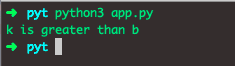That’s it for this tutorial.

This site uses Akismet to reduce spam. Learn how your comment data is processed.